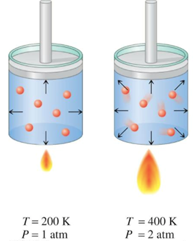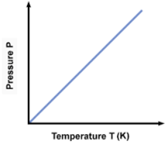# Gay Lussacs Law Formula

## Gay Lussacs Law Formulas - Equation and Problem Solved with Example

The law states that at a constant volume, the pressure (in atm) of a given amount of gas is directly proportional to the temperature (in Kelvin).

Mathematically,

P $\propto$ T at constant volume,V
P/T = k where k is a constantWhich implies,
P1 / T1 = P2 / T2
Or graphically,
When pressure of the gas is plotted against temperature at constant volume, we would obtain a straight line passing through the origin. At any point on the graph the ratio of P : T will always be constant.Example: A 30.0 L sample of nitrogen inside a rigid, metal container at 20.0 °C is placed inside an oven whose temperature is 50.0 °C. The pressure inside the container at 20.0 °C was at 3.00 atm. What is the pressure of the nitrogen after its temperature is increased to 50.0 °C?
Solution:
From Gay Lussac’s Law we know that at constant volume (30 L in this question),
P1 / T1 = P2 / T2
Convert given temperature in degree Celsius to Kelvin
T1 = 20 + 273
T1 = 293 K

T2 = 50 + 273
T2 = 323 K
Let, P1 = 3.00 atm and P2 = x;
Then,
3 / 293 = x / 323
Which implies,
x = 3.31 atm

Question: A gas has a pressure of 699.0 mmHg at 40.0 °C. What is the temperature at standard pressure
Options:
(a) 310 K
(b) 380 K
(c) 400 K
(d) 340 K